# Martynov Sarkisov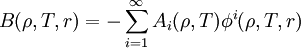$B(\rho, T, r)= - \sum_{i=1}^\infty A_i (\rho,T) \phi^i (\rho, T, r)$
where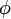$\phi$ is the chosen basis function and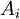$A_i$ are the coefficients determined from thermodynamic consistency conditions. The Martynov-Sarkisov closure is based on the expansion of the bridge function in powers of the thermal potential.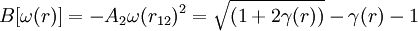$B[\omega(r)]= - A_2 \omega(r_{12})^2 = \sqrt{(1+2\gamma(r))}-\gamma(r) -1$
where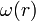$\omega(r)$ is the thermal potential and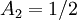$A_2=1/2$. (This closure formed the basis for the Ballone-Pastore-Galli-Gazzillo closure for hard sphere mixtures). Charpentier and Jaske  have observed that the value of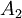$A_2$ differs drastically from 0.5 for temperatures greater than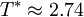$T^*\approx 2.74$, thus the Martynov-Sarkisov closure is deficient in the supercritical domain.